Courses

# Methods of Interpolation - Interpolation and Extrapolation, Business Mathematics and Statistics B Com Notes | EduRev

Created by: Arshit Thakur

## B Com : Methods of Interpolation - Interpolation and Extrapolation, Business Mathematics and Statistics B Com Notes | EduRev

The document Methods of Interpolation - Interpolation and Extrapolation, Business Mathematics and Statistics B Com Notes | EduRev is a part of the B Com Course Business Mathematics and Statistics.
All you need of B Com at this link: B Com

Introduction

We discuss here a number of interpolation methods that we commonly nd in computer graphics and geometric modeling. Interpolation means to calculate a point or several points between two given points. For a given sequence of points, this means to estimate a curve that passes through every single point.

Linear Interpolation

Linear interpolation is the simplest interpolation method. Applying linear interpolation to a sequence of points results in a polygonal line where each straight line segment connects two consecutive points of the sequence. Therefore, every segment (P; Q) is interpolated independently as follows:

P (t) = (1 t) :P + t :Q                               (1)

where t ∈ [0; 1]. By varying t from 0 to 1 we get all the intermediate points between P and Q. Note that P (t) = P for t = 0 and P (t) = Q for t = 1. For values of t < 0 and t > 1 result in extrapolation, that is, we get points on the line de ned by P , Q, but outside the segment (P; Q).

Cosine Interpolation

As shown in Fig. ??, the curve resulting from Linear interpolation has discontinuities at each point. In certain circumstances, we need a smoother interpolating function, that is a function that allows for a smooth transition between consecutive segments. The cosine interpolation carries out a transition that looks smooth, though every segment is interpolated independently .

Cubic Interpolation
...

Hermite Interpolation
...

Linear Interpolation Let us now de ne linear interpolation in more mathematical terms.
De nition 1. A linear interpolation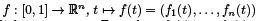is an affine transformation from an unit interval [0; 1] to a straight line segment in Rn , where f1 (t); : : : ; fn (t) are the function components of f along each coordinate axis.
See Lecture 1 for more details on affine transformations. By de nition, an affine transformation preserves barycentric combinations. Therefore, if t ∈ [0; 1] is de ned as a barycentric combination of the points 0; 1 ∈ R

t = α0 :0 + α1 :1; with α0 + α1 = 1

then,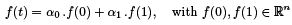with α0 = 1 - t and α1 = t. This is illustrated in Fig.??, where we intuitively see that the linear interpolation preserves the ratio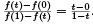Linear Interpolation and Barycentric Coordinates

Let us rst see the relation between collinearity and barycentric coordinates. Let P0 , P , P1 be three collinear points in R3 . Then, P is the barycentric combination of P1 and P2 given as follows: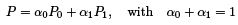where  α0 and  α1 are the barycentric coordinates of P with respect to P0 and P1 , that is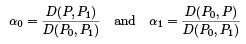where D(; ) denotes the signed Euclidean distance between two points.
We are now at a position that allows to show that the linear interpolation is given by Eq. (1). Taking into consideration the above expressions for α0 and α1 , and the fact that a linear interpolation preserves barycentric coordinates, we have: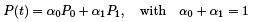where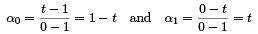that is,

P (t) = (1 t) :P0 + t :P1

Linear Interpolation and Geometric Ratios

By de nition, the ratio of three collinear points P0 , P , and P1 is given by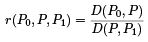Taking into account the expressions of the barycentric coordinates α0 , 1 given above, we have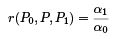We know that an affine transformation f : [0; 1] → Rn preserves barycentric coordinates; as a consequence, the ratio of barycentric coordinates is also preserved. Therefore, r(P0 ; P; P1 ) remains unchanged by affine transformations, that is,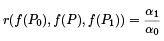In short, an affine transformation preserves the geometric ratio of collinear points, that is, the image of a straight line segment is a straight line segment.

Linear Interpolation over [a; b]

The interval [a; b] can be obtained from the affine transformation of the interval [0; 1]. With t ∈ [0; 1] and u∈ [a; b], this affine trnsformation is given by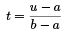so, replacing the expression of t into

P (t) = (1 t)P0 + tP1

we have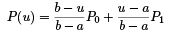Because a; u; b and 0; t; 1 have the same geometric ratio as P0 ; P; P1 , we end up showing that the linear interpolation is invariant under affine domain mappings. By affine domain mapping we mean an affine transformation from the real line to itself.

Piecewise Linear Interpolation

Piecewise linear interpolation involves not two points but a sequence of points P0 ; P1 ; : : : ; PN ∈ Rn such that a linear interpolation is applied to two consecutive points of this sequence. The result is a polyline P, called piecewise linear interpolant of all points P0 ; P1 ; : : : ; PN . This is illustrated in FIg. ??, where we determine a point in each segment for every t ∈ [0; 1].
If the points P0 ; P1 ; : : : ; PN are on a curve C , we say that the resulting polyline P is a piecewise linear interpolant of the curve C ; symbolically, we write P = P(C ).
The piecewise linear interpolation enjoys two properties, as described in the sequel.

Property L4.1 (affine Invariance )

If a curve C is sub ject to an affine transformation f , then a piecewise linear interpolant of f (C ) is an affine transformation of the original piecewise linear interpolant, that is,

P(f (C )) = f (P(C ))

Property L4.2 (Variance Diminishing )

Let P(C ) be a piecewise linear interpolant of the curve C , and π an arbitrary hyperplane that intersects both C and P(C ). Then, we have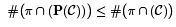that is, the number of intersection points between the plane  and the interpolant is less or equal to the number of points resulting from the intersection between  and the curve.
This is so because, unlike a straight line segment of the interpolant, the curve segment passing through the two endpoints of such a straight line segment is not necessarily convex.

The Menelaus Theorem

Let us now have a look at an important theorem in the context of piecewise linear interpolation.
Theorem 2. Let A; B ; C ∈ R2 be three points de ning two straight lines that meet at B , and D; E ; F points in the lines de ned by (B ; C ), (A; C ), and (A; B ), respectively, each one of which is distinct from the vertices of the triangle ΔAB C . Then, the points D; E ; F are said to be collinear if and only if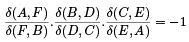Proof Let us consider the piecewise linear interpolant of the points P0 ; P1 ; P2 . Let us apply the same linear interpolation to two points t; u ∈ [0; 1] ⊂ R in a way we get two image points P (t); P (u) in the straight line segment (P0 ; P1 ), and other two image points Q(t); Q(u) in the straight line segment (P1 ; P2 ) in R2 , as illustrated in Fig. ??.

We intend to prove that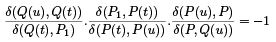For that purpose, we have only to determine the unknown third ratio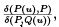, that is, we have to determine the barycentric coordinates of P . Taking into account that P is a barycentric combination of both straight line segments (P (u); Q(u)) and (P (t); Q(t)), we have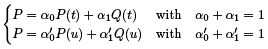(2)

Now, let us substitute the expressions of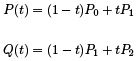and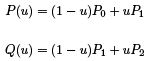into (7) so that we get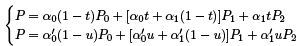(3)

By combining (7) and ((3)), we have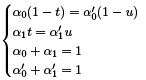(4)

that is,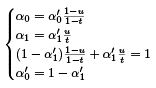(5)

or, equivalently,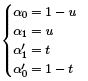(6)

Substituting these barycentric coordinates in (7), we obtain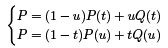(7)

Finally, we can write down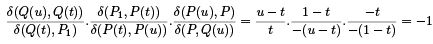Repeated Linear Interpolation

Let us now see how repeated linear interpolation allows for a procedure to construct parabolas. As we will see in lectures to come, the generalization of this procedure leads us to the construction of B'ezier curves.
So, let P0 ; P1 ; P2 be three points in R2 . Using piecewise linear interpolation, we determine two points for a given t ∈ R, one in the straight line de ned by (P0 ; P1 ) and another de ned by (P1 ; P2 ), as follows: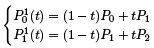(8)

where the exponent 1 indicates the degree of the polynomial. By applying the same linear interpolation at t ∈ R to the new segment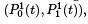, we have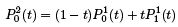(9)

Substituting the expressions of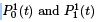given by (8) into (9), we obtain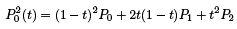(10)

which is a degree-2 polynomial representing a parabola. This construction procedure for parabolas uses repeated linear interpolation.

Property L4.3 (Convex Hull )

The construction of a parabola using repeated linear interpolation enjoys the following the convex hull property, because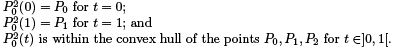Property L4.4 (Affine Invariance )

Taking into consideration the ratios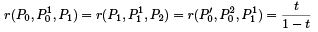we conclude that the above construction of a parabola is invariant under affine transformations because the piecewise linear interpolation is affine invariant.

122 videos|142 docs

,

,

,

,

,

,

,

,

,

,

,

,

,

,

,

,

,

,

,

,

,

,

,

,

;